# generate X & Y coordinates into table## Recommended PostsI working on urban planning drawings where i work on huge numbers of villas everyday. All the property limit of villas are POLYLINE. I'am taking X & Y coordianteseach of corner of the polyline (villa) one by one & pasting it into table (see the image) ...i know this is a very slow method. Is anybody down there who has the code to make this automatic...i mean by just clicking the POLYLINE & it will automatically gets all the X & Y coordinates (i mean all the corners) & put it automatically on the TABLE.

your help is very much appreciated.

Thanks.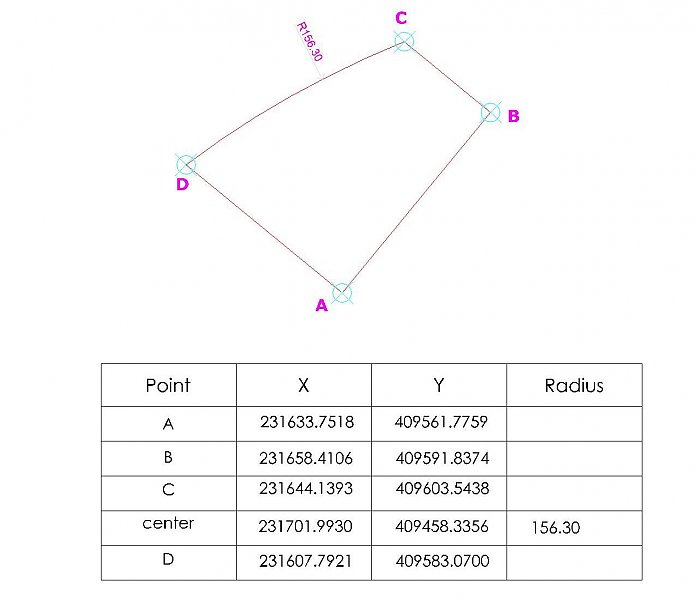• Replies 118
• Created

#### Top Posters In This Topic

•20

•8

•7

•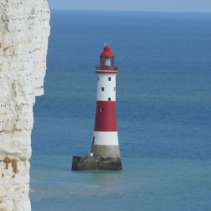7

#### Top Posters In This Topic

•ASMI 20 posts

•Lee Mac 8 posts

•fixo 7 posts

•eldon 7 posts

#### Popular PostsGive that a try   ~'J'~ CoordsToTable.LSP> The Courage Dog & jason tay   There is new version with some improvements.   'Letters' version for Courage Dog:   (defun c:tabord(/ aCen cAng cCen cPl cRad cReg fDr it lCnt lLst mSp

#### Posted ImagesGive that a try

~'J'~

CoordsToTable.LSP

•1
##### Share on other sitesthanks Fixo, i'll try it.

##### Share on other sitesGive that a try

~'J'~

I try your code & it didn't work. there's an error saying: ; error: AutoCAD.Application: AcRxClassName entry is not in the system registry

what does it mean?

##### Share on other sites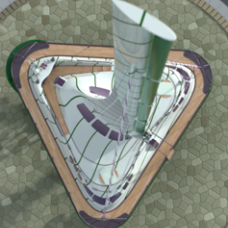I’m not sure about the source of the error but I can say, once that issue is sorted out, you will be quite pleased with the performance of the routine. Nice work Fixo.

##### Share on other sitesI try your code & it didn't work. there's an error saying: ; error: AutoCAD.Application: AcRxClassName entry is not in the system registry

what does it mean?

What the Acad version you uses?

Guess the problem is with 'AutoCAD.AcCmColor' object

```(defun add-table-style (adoc / ;|acmcol|; adoc clsname keyname newstyleobj tbldict tblstylename)

(setq	tbldict
(vla-item
(vla-get-dictionaries
)
)
)
(setq	keyname	     "NewStyle"
clsname    "AcDbTableStyle"
tblstylename "Coordinates" ;change name
)
(setq	newstyleobj
)
(vlax-put newstyleobj 'TitleSuppressed :vlax-false)
;;;  (setq	acmcol (vla-GetInterfaceObject
;;;	       )
;;;  )
;;;  (vlax-put acmcol 'Colorindex 24)
(vlax-put newstyleobj 'Name TblStyleName)
(vlax-put newstyleobj 'Description "Coordinates Table")
(vlax-put newstyleobj 'BitFlags 1)
(vlax-put newstyleobj 'HorzCellMargin 0.06)
(vlax-put newstyleobj 'VertCellMargin 0.06)

;;;  (vlax-invoke newstyleobj 'SetColor acDataRow acmcol)
(vlax-invoke newstyleobj
'SetBackgroundColorNone
acDataRow
:vlax-false
)
(vlax-invoke newstyleobj 'SetTextStyle acDataRow "Standard")
;;;  (vlax-invoke newstyleobj 'SetTextHeight acTitleRow 0.25)
(vlax-invoke newstyleobj 'SetTextHeight acDataRow 0.18)
(vlax-invoke newstyleobj 'SetGridVisibility acVertInside acDataRow
:vlax-true)
(vlax-invoke newstyleobj
'SetAlignment
acDataRow
acMiddleCenter
)
(vla-update newstyleobj)
;;;  (vlax-release-object acmcol)
;| ETC |;
(princ)
)```

~'J'~

##### Share on other sitesI’m not sure about the source of the error but I can say, once that issue is sorted out, you will be quite pleased with the performance of the routine. Nice work Fixo.

Hi, SEANT

Thanks for the kind words

Happy computing~'J'~

##### Share on other sites> The Courage Dog

I have not forgotten about you. Simply it has borrowed 2 hours of time per quiet conditions. But quiet conditions was not. I feel that on this site is time to take holiday... Another one, text size depends on TEXTSIZE sysem variable (and table dimensions also):

```(defun c:tabord(/ aCen cAng cCen cPl cRad cReg
fDr it lCnt lLst mSp pCen pT1
pT2 ptLst R tHt tLst vlaPl vlaTab
vLst cTxt oldCol nPl clFlg *error*)

(defun Extract_DXF_Values(Ent Code)
(mapcar 'cdr
(vl-remove-if-not
'(lambda(a)(=(car a)Code))
(entget Ent)))
); end of

(defun *error*(msg)
(setvar "CMDECHO" 1)
(princ)
); end of *error*

(if
(and
(setq cPl(entsel "\nSelect LwPoliline > "))
(= "LWPOLYLINE"(car(Extract_DXF_Values(car cPl)0)))
); end and
(progn
(setq vlaPl(vlax-ename->vla-object(car cPl))
ptLst(mapcar 'append
(setq vLst(Extract_DXF_Values(car cPl)10))
(mapcar 'list(Extract_DXF_Values(car cPl)42)))
lLst '("A" "B" "C" "D" "E" "F" "G" "H" "I" "J" "K" "L" "M"
"N" "O" "P" "Q" "R" "S" "T" "U" "V" "W" "X" "Y" "Z")
r 2 lCnt 0
tLst '((1 0 "Point")(1 1 "X")(1 2 "Y")(1 3 "Radius"))
mSp(vla-get-ModelSpace
(vla-get-ActiveDocument
tHt(getvar "TEXTSIZE")
); end setq
(setvar "CMDECHO" 0)
(foreach vert ptLst
(setq vert(trans vert 0 1)
tLst(append tLst
(list(list r 0 (nth lCnt lLst))
(list r 1(rtos(car vert)2 4))
(list r 3 ""))))
(if(and
(/= 0.0(last vert))
(setq pt1(vlax-curve-GetPointAtParam vlaPl lCnt))
(setq pt2(vlax-curve-GetPointAtParam vlaPl(1+ lCnt)))
); end and
(setq r(1+ r)
2(sin(/(* 4(atan(abs(last vert))))2))))
aCen(vlax-curve-GetPointAtParam vlaPl(+ 0.5 lCnt))
fDr(vlax-curve-getFirstDeriv vlaPl
(vlax-curve-getParamAtPoint vlaPl aCen))
pCen(trans
(polar aCen(-(if(minusp(last vert)) pi(* 2 pi))
tLst(append tLst(list
(list r 0 "center")
(list r 1(rtos(car pCen)2 4))
(list r 3(rtos cRad 2 4))))
); end setq
); end if
(setq r(1+ r) lCnt(1+ lCnt))
); end foreach
(setq vlaTab(vla-AddTable mSp (vlax-3D-point '(0 0 0))
(+ 1(/(length tLst)4)) 4 (* 3 tHt)(* 18 tHt)))
(foreach i tLst
(vl-catch-all-apply 'vla-SetText(cons vlaTab i))
); end foreach
(vla-DeleteRows vlaTab 0 1)
(princ "\n<<< Place Table >>> ")
(command "_.copybase" (trans '(0 0 0)0 1)(entlast) "")
(command "_.erase" (entlast) "")
(command "_.pasteclip" pause)
(if(= :vlax-true(vla-get-Closed vlaPl))
(progn
(setq nPl(vla-Copy vlaPl))
(command "_.region" (entlast) "")
(setq cCen(vlax-get(setq cReg
(vlax-ename->vla-object(entlast)))'Centroid))
(vla-Delete cReg)
(setq clFlg T)
); end progn
); end if
(setq lCnt 0)
(foreach v vLst
(if clFlg
(setq cAng(angle cCen(trans v 0 1))
iPt(polar v cAng (* 2 tHt)))
(setq fDr(vlax-curve-getFirstDeriv vlaPl
(vlax-curve-getParamAtPoint vlaPl v))
iPt(trans
(polar v(-(* 2 pi)(atan(/(car fDr)(cadr fDr))))
(* 2 tHt))0 1)
); end if
); end if
(vlax-3d-point iPt) tHt)
lCnt(1+ lCnt)
); end setq
(setq oldCol(getvar "CECOLOR"))
(setvar "CECOLOR" "1")
(command "_.circle" v (/ tHt 3))
(setvar "CECOLOR" oldCol)
); end foreach
(setvar "CMDECHO" 1)
); end progn
(princ "\n<!> It isn't LwPolyline! Quit. <!> ")
); end if
(princ)
); end of c:tabord```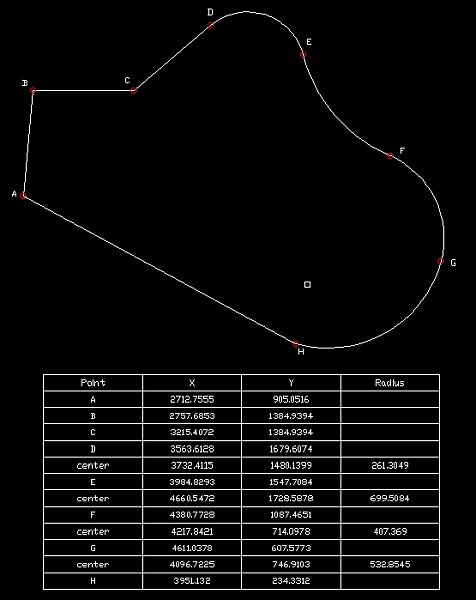##### Share on other sitesIn code above was one bug. Now it works ok.

##### Share on other sitesyou are a real genius, this code is fantastic....this is exactly what i'm lookingfor.....thanks Asmi.

Thanks to you also Mr. Fixo

##### Share on other sites##### Share on other sitesAsmi i tried your super powerful code, it works very fine...is it possible to set the height & width of cells & set the text style into ARIAL with 2.5 height & .90 width factor?

your code really help me a lot...

thanks

the courage dog

##### Share on other sitesOf course I can to make it. But... I not so wish to make it as it reduces universality of the program. Probably other users do not wish to use Arial. You can make text style with font Arial and width factor 0.9 and table style with this text style. In this case all will work as you want.

Concerning height and width of cells. It too is possible. At present the height and width depend on current value of variable TEXTSIZE. I can or add inquiries of height and width or to change proportions of dependence from TEXTSIZE. This way seems to me to more correct. Publish one more picture with demanded proportions.

If inquiries of height are necessary and width of cells I also am able to do it. Cell width for every column do you thing? I thing - too many requests##### Share on other sitesMay be other data as Area, Perimetre etc. if "Add additional data [Yes/Not]: " ?

##### Share on other sitesDear ASMI..the code really great..just to ask how to change the point

to numbering which we dont have a limit

because the method i use will have and limit as shown on below

lLst '("1" "2" "3" "4" "5" "6" "7" "8" "9" "10" "11" "12" "13"

"14" "15" "16" "17" "18" "19" "20" "21" "22" "23" "24" "25" "26" "27" "28" "29" "30" "31" "32")

and i find that if that the label seem to stop when come to 0degree, 90 degree or 180 degree(if the line is not a close line)..

Hope to learn from you, and i will alway keep reading on your

web page www.asmitools.com##### Share on other sites> jason tay

Come tomorrow Jason. I have urgent job today and havn't time alternate my code. Thank you for bug catching, I guess it can be 'Divide to 0', I try to fix it tomorrow.

##### Share on other sites> The Courage Dog & jason tay

There is new version with some improvements.

'Letters' version for Courage Dog:

```(defun c:tabord(/ aCen cAng cCen cPl cRad cReg
fDr it lCnt lLst mSp pCen pT1
pT2 ptLst R tHt tLst vlaPl vlaTab
vLst cTxt oldCol nPl clFlg actDoc
tPt1 tPt2 cAng tiPt oSnp *error*)

(defun Extract_DXF_Values(Ent Code)
(mapcar 'cdr
(vl-remove-if-not
'(lambda(a)(=(car a)Code))
(entget Ent)))
); end of

(defun *error*(msg)
(setvar "CMDECHO" 1)
(if oSnp(setvar "OSMODE" oSnp))
(if mSp(vla-EndUndoMark actDoc))
(princ)
); end of *error*

(defun Alph_Num(Counter / lLst cRes)
(setq lLst '("A" "B" "C" "D" "E" "F" "G" "H" "I" "J"
"K" "L" "M" "N" "O" "P" "Q" "R" "S" "T"
"U" "V" "W" "X" "Y" "Z"))
(if(<= 1.0(setq cRes(/ Counter 26.0)))
(strcat(itoa(fix cRes))
(nth(- Counter(* 26(fix cRes)))lLst))
(nth Counter lLst)
); end if
); end of Alph_Num

(if
(and
(setq cPl(entsel "\nSelect LwPoliline > "))
(= "LWPOLYLINE"(car(Extract_DXF_Values(car cPl)0)))
); end and
(progn
(setq vlaPl(vlax-ename->vla-object(car cPl))
ptLst(mapcar 'append
(setq vLst(Extract_DXF_Values(car cPl)10))
(mapcar 'list(Extract_DXF_Values(car cPl)42)))
r 2 lCnt 0
tLst '((1 0 "Point")(1 1 "X")(1 2 "Y")(1 3 "Radius"))
actDoc(vla-get-ActiveDocument
mSp(vla-get-ModelSpace actDoc)
tHt(getvar "TEXTSIZE")
); end setq
(setvar "CMDECHO" 0)
(setq oSnp(getvar "OSMODE"))
(vla-StartUndoMark actDoc)
(foreach vert ptLst
(setq vert(trans vert 0 1)
tLst(append tLst
(list(list r 0(Alph_Num lCnt))
(list r 1(rtos(car vert)2 4))
(list r 3 ""))))
(if(and
(/= 0.0(last vert))
(setq pt1(vlax-curve-GetPointAtParam vlaPl lCnt))
(setq pt2(vlax-curve-GetPointAtParam vlaPl(1+ lCnt)))
); end and
(setq r(1+ r)
2(sin(/(* 4(atan(abs(last vert))))2))))
aCen(vlax-curve-GetPointAtParam vlaPl(+ 0.5 lCnt))
fDr(vlax-curve-getFirstDeriv vlaPl
(vlax-curve-getParamAtPoint vlaPl aCen))
pCen(trans
(polar aCen(-(if(minusp(last vert)) pi(* 2 pi))
tLst(append tLst(list
(list r 0 "center")
(list r 1(rtos(car pCen)2 4))
(list r 3(rtos cRad 2 4))))
); end setq
); end if
(setq r(1+ r) lCnt(1+ lCnt))
); end foreach
(setq vlaTab(vla-AddTable mSp (vlax-3D-point '(0 0 0))
(+ 1(/(length tLst)4)) 4 (* 3 tHt)(* 20 tHt)))
(foreach i tLst
(vl-catch-all-apply 'vla-SetText(cons vlaTab i))
); end foreach
(vla-put-VertCellMargin vlaTab (* 0.75 tHt))
(vla-put-Height vlaTab(* 1.75(/(length tLst)4)))
(vla-SetColumnWidth vlaTab 0 (* 10 tHt))
(vla-SetColumnWidth vlaTab 3 (* 12 tHt))
(vla-put-RepeatTopLabels vlaTab :vlax-true)
(vla-put-BreakSpacing vlaTab (* 3 tHt))
(vla-DeleteRows  vlaTab 0 1)
(princ "\n<<< Place Table >>> ")
(command "_.copybase" (trans '(0 0 0)0 1)(entlast) "")
(command "_.erase" (entlast) "")
(command "_.pasteclip" pause)
(if(= :vlax-true(vla-get-Closed vlaPl))
(progn
(setq nPl(vla-Copy vlaPl))
(command "_.region" (entlast) "")
(setq cCen(vlax-get(setq cReg
(vlax-ename->vla-object(entlast)))'Centroid))
(vla-Delete cReg)
(setq clFlg T)
); end progn
); end if
(setq lCnt 0)
(foreach v vLst
(if clFlg
(setq cAng(angle cCen(trans v 0 1))
iPt(polar v cAng (* 2 tHt)))
(setq tPt1(vlax-curve-GetPointAtParam vlaPl
(- lCnt 0.0000001))
tPt2(vlax-curve-GetPointAtParam vlaPl
(+ lCnt 0.0000001))
iPt(polar v(+(* pi 0.5)(if(minusp
(setq cAng(angle tPt1(if tPt2 tPt2
(polar tPt1(* 0.5 pi)0.0000001)))))
cAng(- cAng)))(* 2 tHt))
); end setq
); end if
(setvar "OSMODE" 0)
(vlax-3d-point iPt) tHt)
tiPt(vla-get-InsertionPoint cTxt)
lCnt(1+ lCnt)
); end setq
(vla-put-Alignment cTxt 10)
(vla-put-TextAlignmentPoint cTxt tiPt)
(setq oldCol(getvar "CECOLOR"))
(setvar "CECOLOR" "1")
(command "_.circle" v (/ tHt 3))
(setvar "CECOLOR" oldCol)
); end foreach
(setvar "OSMODE" oSnp)
(setvar "CMDECHO" 1)
(vla-EndUndoMark actDoc)
); end progn
(princ "\n<!> It isn't LwPolyline! Quit. <!> ")
); end if
(gc)
(princ)
); end of c:tabord```

'Number' version for jason tay:

```(defun c:tabord2(/ aCen cAng cCen cPl cRad cReg
fDr it lCnt lLst mSp pCen pT1
pT2 ptLst R tHt tLst vlaPl vlaTab
vLst cTxt oldCol nPl clFlg actDoc
tPt1 tPt2 cAng tiPt oSnp *error*)

(defun Extract_DXF_Values(Ent Code)
(mapcar 'cdr
(vl-remove-if-not
'(lambda(a)(=(car a)Code))
(entget Ent)))
); end of

(defun *error*(msg)
(setvar "CMDECHO" 1)
(if oSnp(setvar "OSMODE" oSnp))
(if mSp(vla-EndUndoMark actDoc))
(princ)
); end of *error*

(if
(and
(setq cPl(entsel "\nSelect LwPoliline > "))
(= "LWPOLYLINE"(car(Extract_DXF_Values(car cPl)0)))
); end and
(progn
(setq vlaPl(vlax-ename->vla-object(car cPl))
ptLst(mapcar 'append
(setq vLst(Extract_DXF_Values(car cPl)10))
(mapcar 'list(Extract_DXF_Values(car cPl)42)))
r 2 lCnt 0
tLst '((1 0 "Point")(1 1 "X")(1 2 "Y")(1 3 "Radius"))
actDoc(vla-get-ActiveDocument
mSp(vla-get-ModelSpace actDoc)
tHt(getvar "TEXTSIZE")
); end setq
(vla-StartUndoMark actDoc)
(setvar "CMDECHO" 0)
(setq oSnp(getvar "OSMODE"))
(foreach vert ptLst
(setq vert(trans vert 0 1)
tLst(append tLst
(list(list r 0(itoa(1+ lCnt)))
(list r 1(rtos(car vert)2 4))
(list r 3 ""))))
(if(and
(/= 0.0(last vert))
(setq pt1(vlax-curve-GetPointAtParam vlaPl lCnt))
(setq pt2(vlax-curve-GetPointAtParam vlaPl(1+ lCnt)))
); end and
(setq r(1+ r)
2(sin(/(* 4(atan(abs(last vert))))2))))
aCen(vlax-curve-GetPointAtParam vlaPl(+ 0.5 lCnt))
fDr(vlax-curve-getFirstDeriv vlaPl
(vlax-curve-getParamAtPoint vlaPl aCen))
pCen(trans
(polar aCen(-(if(minusp(last vert)) pi(* 2 pi))
tLst(append tLst(list
(list r 0 "center")
(list r 1(rtos(car pCen)2 4))
(list r 3(rtos cRad 2 4))))
); end setq
); end if
(setq r(1+ r) lCnt(1+ lCnt))
); end foreach
(setq vlaTab(vla-AddTable mSp (vlax-3D-point '(0 0 0))
(+ 1(/(length tLst)4)) 4 (* 3 tHt)(* 20 tHt)))
(foreach i tLst
(vl-catch-all-apply 'vla-SetText(cons vlaTab i))
); end foreach
(vla-put-VertCellMargin vlaTab (* 0.75 tHt))
(vla-put-Height vlaTab(* 1.75(/(length tLst)4)))
(vla-SetColumnWidth vlaTab 0 (* 10 tHt))
(vla-SetColumnWidth vlaTab 3 (* 12 tHt))
(vla-put-RepeatTopLabels vlaTab :vlax-true)
(vla-put-BreakSpacing vlaTab (* 3 tHt))
(vla-DeleteRows  vlaTab 0 1)
(princ "\n<<< Place Table >>> ")
(command "_.copybase" (trans '(0 0 0)0 1)(entlast) "")
(command "_.erase" (entlast) "")
(command "_.pasteclip" pause)
(if(= :vlax-true(vla-get-Closed vlaPl))
(progn
(setq nPl(vla-Copy vlaPl))
(command "_.region" (entlast) "")
(setq cCen(vlax-get(setq cReg
(vlax-ename->vla-object(entlast)))'Centroid))
(vla-Delete cReg)
(setq clFlg T)
); end progn
); end if
(setq lCnt 0)
(foreach v vLst
(if clFlg
(setq cAng(angle cCen(trans v 0 1))
iPt(polar v cAng (* 2 tHt)))
(setq tPt1(vlax-curve-GetPointAtParam vlaPl
(- lCnt 0.0000001))
tPt2(vlax-curve-GetPointAtParam vlaPl
(+ lCnt 0.0000001))
iPt(polar v(+(* pi 0.5)(if(minusp
(setq cAng(angle tPt1(if tPt2 tPt2
(polar tPt1(* 0.5 pi)0.0000001)))))
cAng(- cAng)))(* 2 tHt))
); end setq
); end if
(setvar "OSMODE" 0)
(vlax-3d-point iPt) tHt)
tiPt(vla-get-InsertionPoint cTxt)
lCnt(1+ lCnt)
); end setq
(vla-put-Alignment cTxt 10)
(vla-put-TextAlignmentPoint cTxt tiPt)
(setq oldCol(getvar "CECOLOR"))
(setvar "CECOLOR" "1")
(command "_.circle" v (/ tHt 3))
(setvar "CECOLOR" oldCol)
); end foreach
(setvar "OSMODE" oSnp)
(setvar "CMDECHO" 1)
(vla-EndUndoMark actDoc)
); end progn
(princ "\n<!> It isn't LwPolyline! Quit. <!> ")
); end if
(gc)
(princ)
); end of c:tabord2```

•1
##### Share on other sitesA very useful routine, thank you ASMI.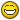:thumbsup:I have been trying out the "number" version, and I noticed a couple of differences from the original. The table goes to 0,0 instead of being able to pick the position, and no circles and numbers are drawn by each vertex.

Two insignificant bugs, but I can't spot what code makes it different.

##### Share on other sitesYes, I fogot one thing. Now should works ok.

##### Share on other sitesI am confused, I can't get it to work as it was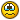I am using 2005, so it might be a version difference.

ASMI, could you please put a header in your programmes with your name and the date and time of the latest version? Then in years to come, we can remember the author of these very useful routines. Thank you## Join the conversation

You can post now and register later. If you have an account, sign in now to post with your account.
Note: Your post will require moderator approval before it will be visible.×   Pasted as rich text.   Restore formatting

Only 75 emoji are allowed.